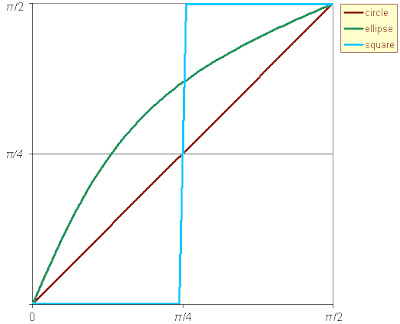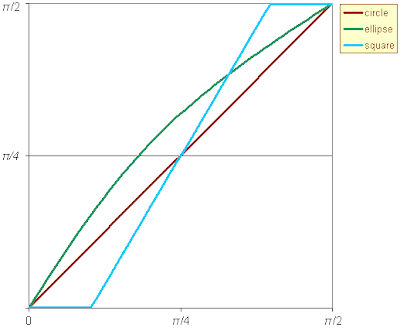## Saturday, December 8, 2007

### Goal crossbar cross-sections

In the game of soccer, it is not infrequent that the ball hits the goal crossbar: sometimes it bounces back into the field or out over the bar, and sometimes one's lucky and the ball enters the goal.The result depends on the point of the crossbar where the hit occurs and the incidence angle of the ball. The question arises: how does the bar cross-section affect the chances that the ball bounces into the goal? Although goals at professional fields seem to always have crossbars with circular cross-section, the FIFA Laws of the Game allow for other shapes:

Goalposts and crossbars must be made of wood, metal or other approved material. Their shape may be square, rectangular, round or elliptical and they must not be dangerous to players.

The geometry involved is simple: Let α be the incidence angle of the ball and η the inclination of the crossbar surface at the hitting point. As depicted in the diagram, negative values of α would mean that the ball follows an ascending trajectory.The reflection angle γ, measured clockwise from 12 o'clock, can be easily calculated:

γ = π/2 + 2η + α.

The ball enters the goal when γ > π, hence the minimum incidence angle for which the ball enters the goal at a particular hitting point is

αgoal = π/2 − 2η.

We now traverse the crossbar section in polar coordinates (ρ,θ), with θ growing clockwise from 3 o'clock, and determine the incidence angle η as a function of θ.For a circular cross-section, η = θ; if the section is an ellipse with eccentricity ε then η = arctan((1−ε2)−1 tan θ), whereas for a rectangle η(θ) consists of two constant segments corresponding to the the vertical and horizontal edges.The analysis so far considers an idealized ball with null radius. This approximation is grossly incorrect in the particular case of a rectangular cross-section, as it fails to model the situation where the ball hits on an edge of the crossbar rather than a flat face. An easy trick to cope with a ball radius r > 0 is to consider the extended cross-section consisting of those points within distance r of the original section:The extended cross-section for a circle is also a circle, and for an ellipse is something not very different from the original shape (the resulting "eccentricity" is smaller). In the case of a rectangle the abrupt transition between the two values of η(θ) is replaced by a slope which is less steep as the ratio between r and the crossbar width grows; for a square section and considering the typical dimensions of balls and crossbars, the slope value lies around 1.7.We are now ready to depict αgoal as a function of the hitting angle θ and interpret the results:For each hitting angle θ, αgoal is the minimun incidence that the ball trajectory has to have to enter the goal, measuring the incidence angle from the horizontal line downwards. So, lower values of αgoal means that it is easier to enter the goal. For instance, when the hit occurs at low values of θ (front part of the crossbar) the ball has to follow an almost vertical descending trajectory if it is to enter the goal, whereas for high values of θ (bottom part of the bar) even ascending trajectories can bounce into the goal. We see that an elliptical cross-section is always more goal-friendly than a circular section. As for the square section, it is worse (less goal-friendly) than the ellipse for low values of θ and better for a smaller region of high values of θ.

So, what cross-section shape should we choose if we want to facilitate goal scoring? Circles are ruled out, and to decide between an elliptical shape or a square we would have to know which hitting zone is more likely. Although I can't justify it, my hunch is that most hits against the crossbar occur at the front part rather than at the bottom, and moreover the interval of values of θ for which the ellipse wins is larger than the winning interval for the square (the intersection point of both η functions happen at some θ0 > π/4). So, it seems like we should go for an elliptical cross-section, the more eccentric (flatter) the better.